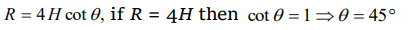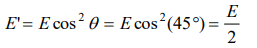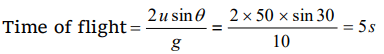## Motion in a Plane Questions and Answers Part-20

1. A projectile thrown with a speed v at an angle $\theta$ has a range R on the surface of earth. For same v and $\theta$ , its range on the surface of moon will be
a) R/6
b) 6R
c) R/36
d) 36R

Explanation:2. The greatest height to which a man can throw a stone is h . The greatest distance to which he can throw it, will be
a) $\frac{h}{2}$
b) h
c) 2h
d) 3h

Explanation:3. The horizontal range is four times the maximum height attained by a projectile. The angle of projection is
a) $90^{\circ}$
b) $60^{\circ}$
c) $45^{\circ}$
d) $30^{\circ}$

Explanation:4. A ball is projected with kinetic energy E at an angle of $45^{\circ}$ to the horizontal. At the highest point during its flight, its kinetic energy will be
a) Zero
b) $\frac{E}{2}$
c) $\frac{E}{\sqrt{2}}$
d) E

Explanation:5. A particle of mass m is projected with velocity v making an angle of 45o with the horizontal. The magnitude of the angular momentum of the particle about the point of projection when the particle is at its maximum height is (where g = acceleration due to gravity)
a) Zero
b) $mv^{3}\diagup \left(4\sqrt{2}g\right)$
c) $mv^{3}\diagup \left(\sqrt{2}g\right)$
d) $mv^{2}\diagup 2g$

Explanation: $mv^{3}\diagup \left(4\sqrt{2}g\right)$

6. A particle reaches its highest point when it has covered exactly one half of its horizontal range. The corresponding point on the displacement time graph is characterised by
a) Negative slope and zero curvature
b) Zero slope and negative curvature
c) Zero slope and positive curvature
d) Positive slope and zero curvature

Explanation: Zero slope and negative curvature

7. At the top of the trajectory of a projectile, the acceleration is
a) Maximum
b) Minimum
c) Zero
d) g

Explanation: Acceleration through out the projectile motion remains constant and equal to g.

8. When a body is thrown with a velocity u making an angle $\theta$ with the horizontal plane, the maximum distance covered by it in horizontal direction is
a) $\frac{u^{2}\sin\theta}{g}$
b) $\frac{u^{2}\sin2\theta}{2g}$
c) $\frac{u^{2}\sin2\theta}{g}$
d) $\frac{u^{2}\cos2\theta}{g}$

Explanation: $\frac{u^{2}\sin2\theta}{g}$

9. A football player throws a ball with a velocity of 50 metre/sec at an angle 30 degrees from the horizontal. The ball remains in the air for $\left(g=10m\diagup s^{2}\right)$
a) 2.5 sec
b) 1.25 sec
c) 5 sec
d) 0.625 sec10. A body of mass 0.5 kg is projected under gravity with a speed of 98 m/s at an angle of $30^{\circ}$ with the horizontal. The change in momentum (in magnitude) of the body is
a) $24.5$  N - s
b) $49.0$  N - s
c) $98.0$  N - s
d) $50.0$  N - s